# Let B = {b1,b2} and C= {(1,62} be bases for R2. Find the change-of-coordinates matrix from...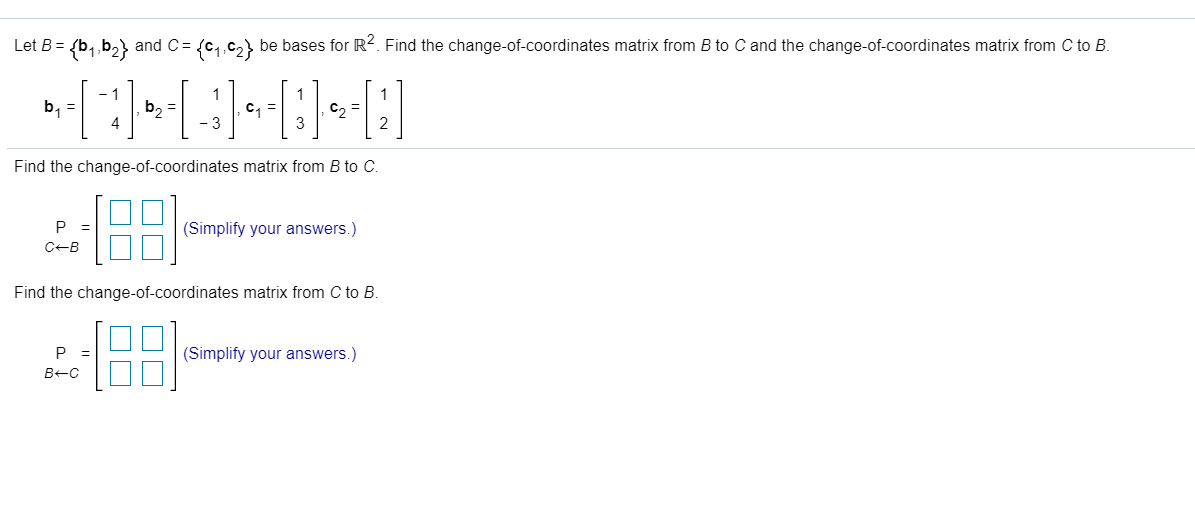Let B = {b1,b2} and C= {(1,62} be bases for R2. Find the change-of-coordinates matrix from B to C and the change-of-coordinates matrix from C to B. - 1 b = b2 = C1 = C = 4 -3 Find the change-of-coordinates matrix from B to C. P = CB (Simplify your answers.) Find the change-of-coordinates matrix from C to B. P B-C [8: (Simplify your answers.)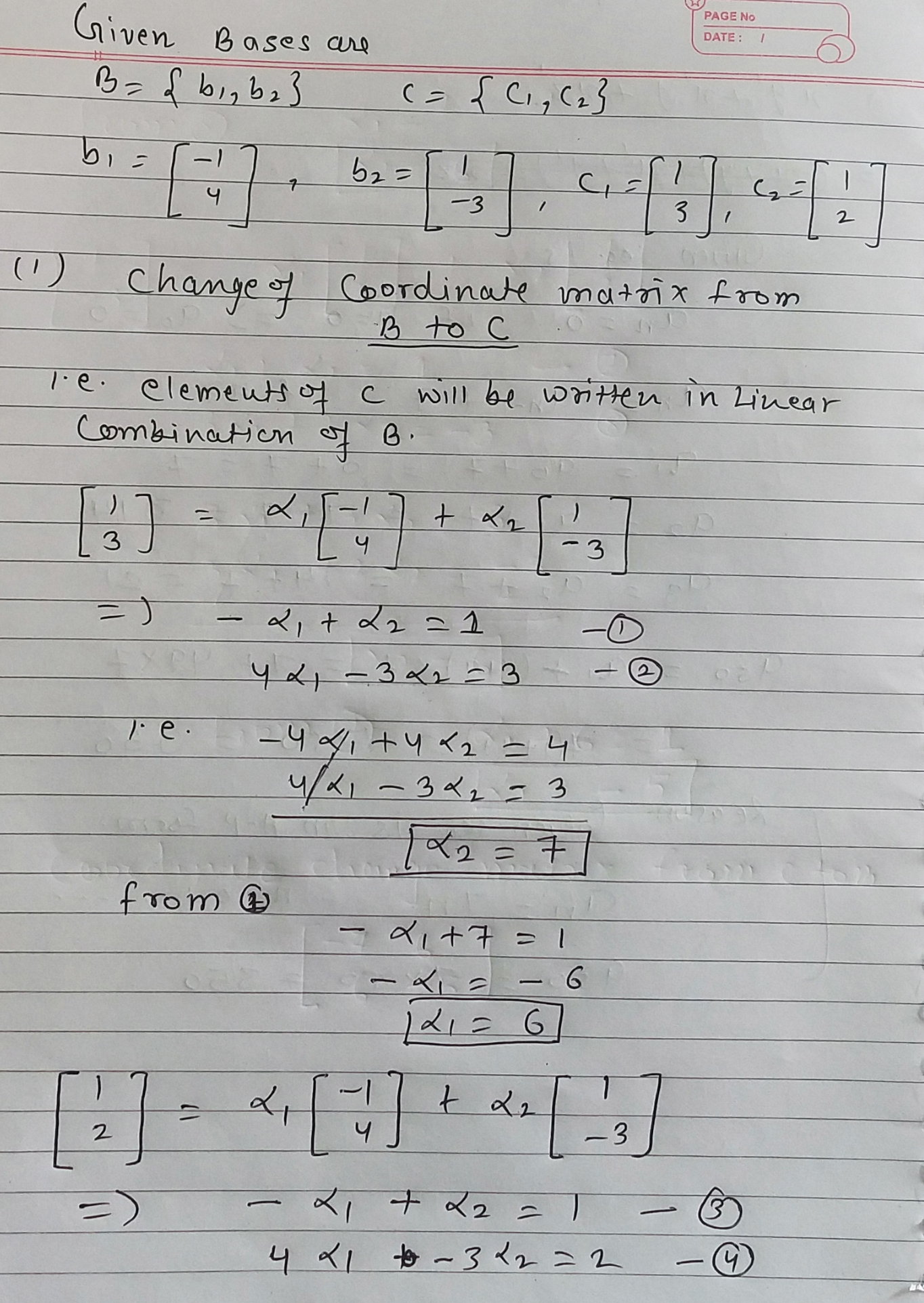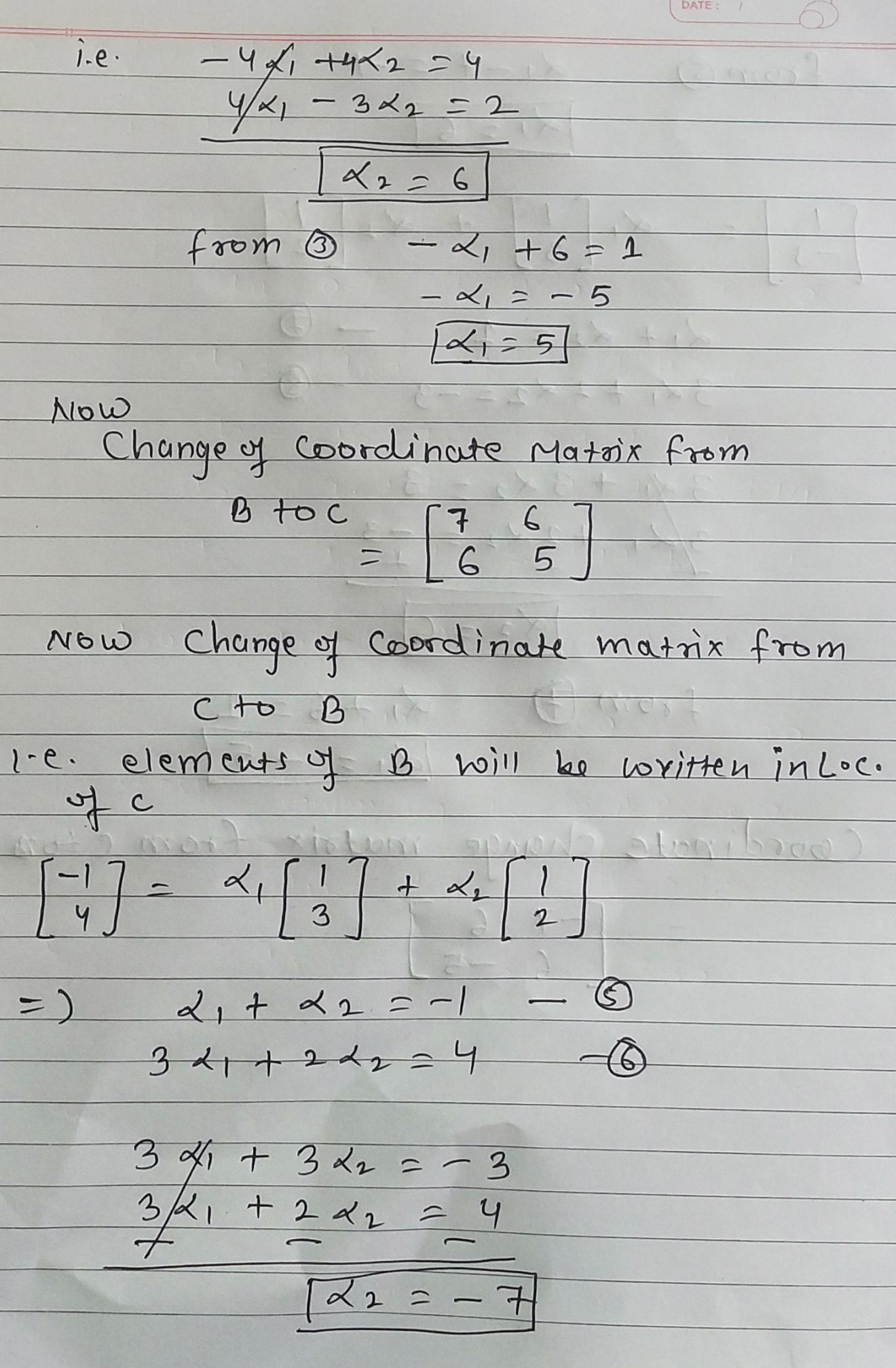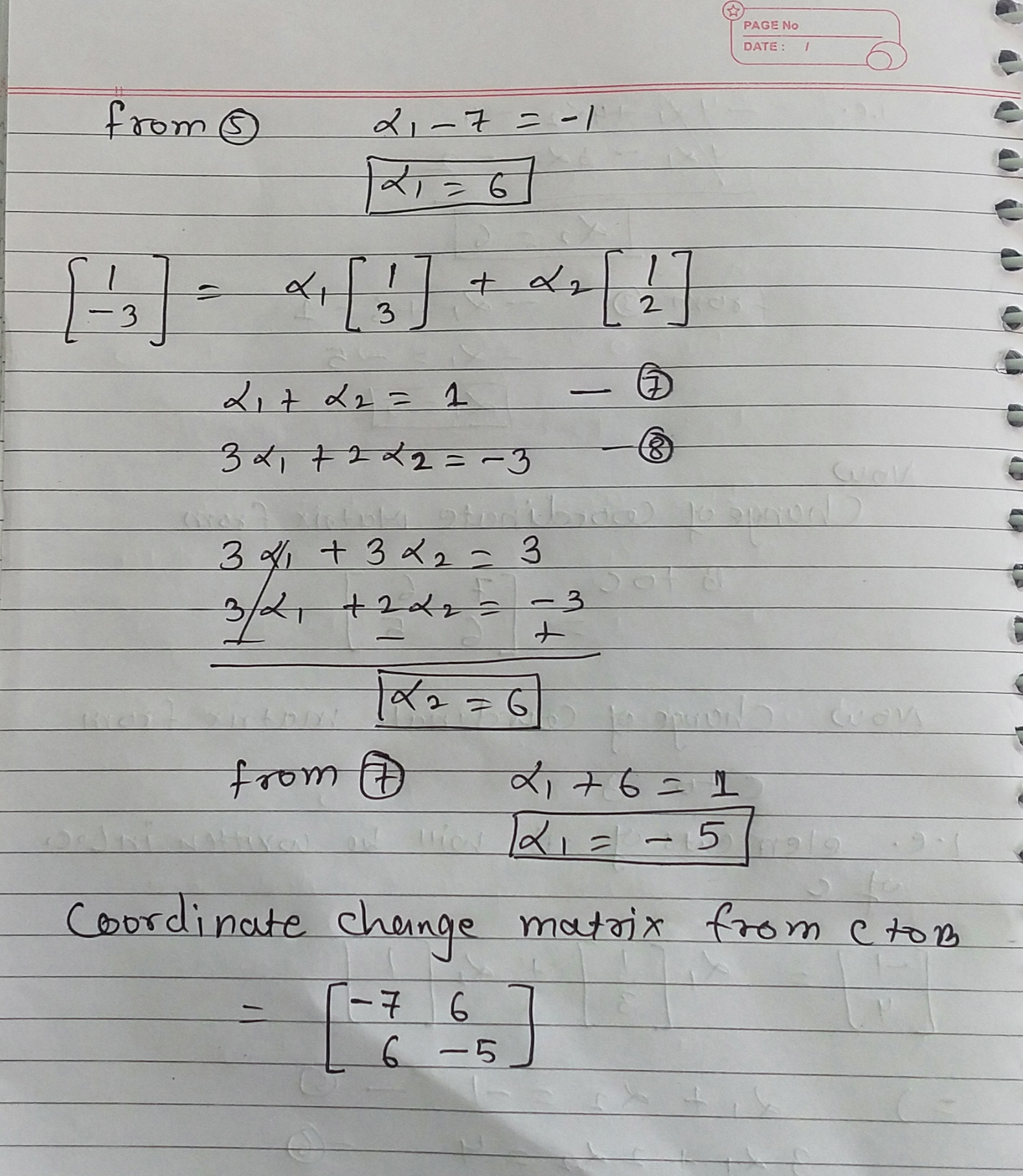#### Earn Coin

Coins can be redeemed for fabulous gifts.

Similar Homework Help Questions
• ### 1) for R2 Given the vectors b1,b2, C1, and cz. B = {b1,b2} is a basis...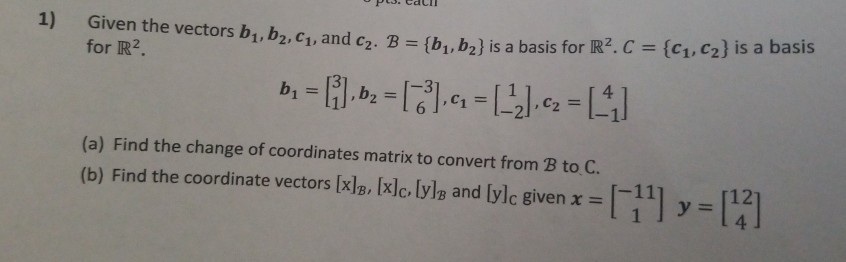1) for R2 Given the vectors b1,b2, C1, and cz. B = {b1,b2} is a basis for R2C = {C1,C2} is a basis b = [i.bz = ,4 = (-2) c2 =  (a) Find the change of coordinates matrix to convert from B to C. (b) Find the coordinate vectors [x]B, [x]c, lyle and [ylc given x =  y = 

• ### Question 2 (10 marks) Consider vectors b) (a) Show that B {bi, b2} and Ć = {ci. C2} are bases for R2 (b) Find the B-coordinates of x- (c) Find the change of coordinates matrix Pc-s from B to C and us...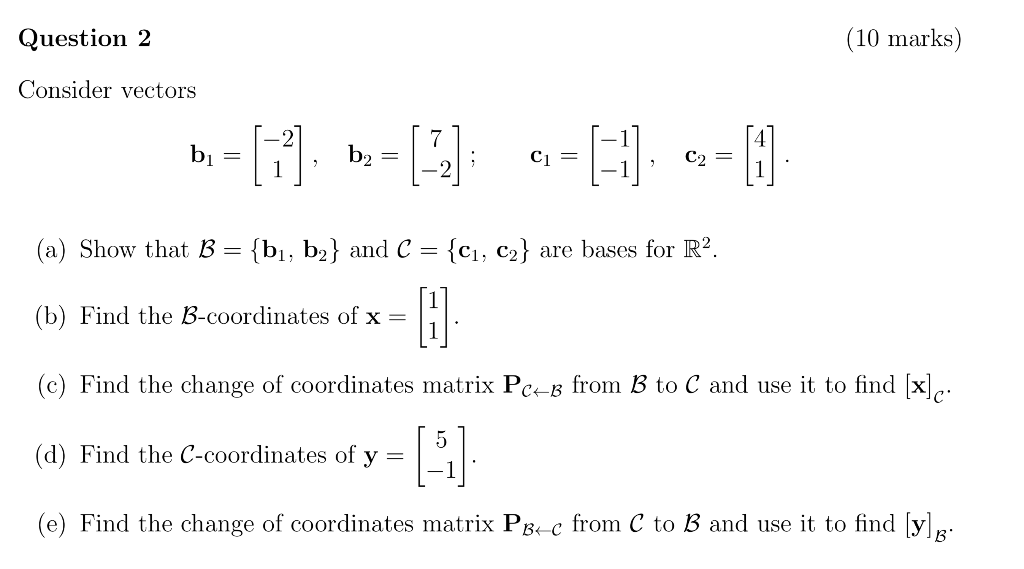Question 2 (10 marks) Consider vectors b) (a) Show that B {bi, b2} and Ć = {ci. C2} are bases for R2 (b) Find the B-coordinates of x- (c) Find the change of coordinates matrix Pc-s from B to C and use it to find [x (d) Find the C-coordinates of y - (e) Find the change of coordinates matrix Psc from C to B and use it to find yg Question 2 (10 marks) Consider vectors b) (a) Show...

• ### Question 6. (15 pts) Let B = {bı, b2} and C = {ci, c2} be bases...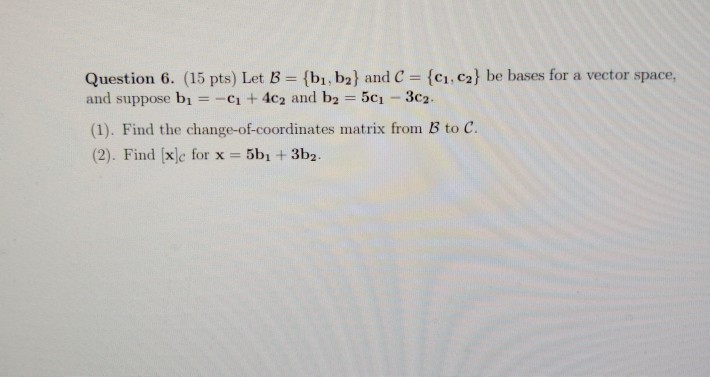Question 6. (15 pts) Let B = {bı, b2} and C = {ci, c2} be bases for a vector space, and suppose bı = - + 4c2 and b2 = 501 - 3c2. (1). Find the change-of-coordinates matrix from B to C. (2). Find [x]c for x = 5bı + 3b2.

• ### Assume that the transition matrix from basis B = {b1, b2, b3} to basis C = {c1, c2, c3} is PC,B = 1/2*[ 0 -1 1 ; -1 1 1 ; 1 0 0 ]. (a) If u = b1 + b2 + 2b3, find [u]C. (b) Calculate PB,C. (c) Suppose...

Assume that the transition matrix from basis B = {b1, b2, b3} to basis C = {c1, c2, c3} is PC,B = 1/2*[ 0 -1 1 ; -1 1 1 ; 1 0 0 ]. (a) If u = b1 + b2 + 2b3, find [u]C. (b) Calculate PB,C. (c) Suppose that c1 = (1, 2, 3), c2 = (1, 2, 0), c3 = (1, 0, 0) and let S be the standard basis for R 3 . (i) Find...

• ### 1. Let B1 = {1, æ, } and B2 = {x - 1,22 - 1 -...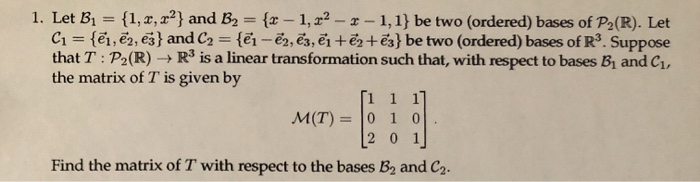1. Let B1 = {1, æ, } and B2 = {x - 1,22 - 1 - 1,1} be two (ordered) bases of P2(R). Let C1 = {ēm, ēm, ēj} and C2 = {@i - ēm, ēz, ?i + ?2+ēz} be two (ordered) bases of R3. Suppose the matrix of T is given by [1 1 1] [2 0 1 Find the matrix of T with respect to the bases B, and C.

• ### Let A = {21,22,23) and B = {b,b2,63} be bases for a vector space V, and...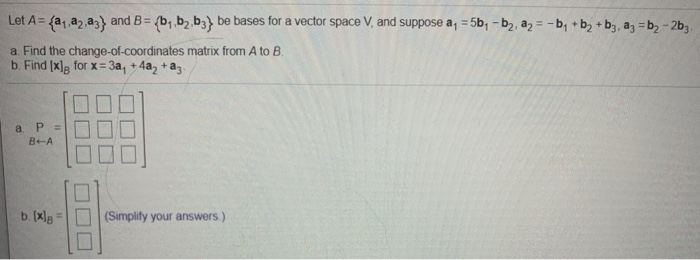Let A = {21,22,23) and B = {b,b2,63} be bases for a vector space V, and suppose a, = 5b, - b», a = -b + b + b3, az = b2 - 253 a Find the change-of-coordinates matrix from A to B. b. Find [x]g for x = 3a + 4a, +az. а PE BA b. [xlg (Simplify your answers.)

• ### (a) Let P(B1∩B2)>0, and A1∪A2⊂B1∩B2. Then show that P(A1|B1).P(A2|B2)=P(A1|B2).P(A2|B1). (b) Let A and B1 be independent;...

(a) Let P(B1∩B2)>0, and A1∪A2⊂B1∩B2. Then show that P(A1|B1).P(A2|B2)=P(A1|B2).P(A2|B1). (b) Let A and B1 be independent; similarly, let A and B2 be independent. Show that in this case, A and B1∪B2 are independent if and only if A and B1∩B2 are independent. (c) Given P(A) = 0.42,P(B) = 0.25, and P(A∩B) = 0.17, find (i)P(A∪B) ; (ii)P(A∩Bc) ; (iii)P(Ac∩Bc) ; (iv)P(Ac|Bc).

• ### Let B = {bį, b2} and C = {C1,C2} be bases for R², where b, -6--0--0--01...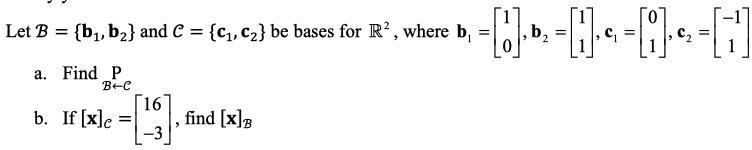Let B = {bį, b2} and C = {C1,C2} be bases for R², where b, -6--0--0--01 1 a. Find P BEC [16 b. If [x]c = -3 de= , find [x]

• ### 5. Let V = P(R). Find the change of coordinates matrix from B = {:22 +2...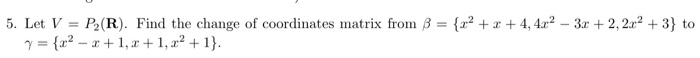5. Let V = P(R). Find the change of coordinates matrix from B = {:22 +2 +4,4x2 – 3x +2, 2x2 + 3} to 7 = {22 - 2+1, 2+1,22 +1}.

• ### 2) Let B = {(1, 3, 4), (2,-5,2), (-4,2-6)) and B/-(( 1, 2,-2), (4, 1,-4), (-2, 5, 8)) be 5 ordered bases of R2. Let x = | 8 | in the standard basis of R2. a) Use a matrix and x to find L18 ]B. b) U...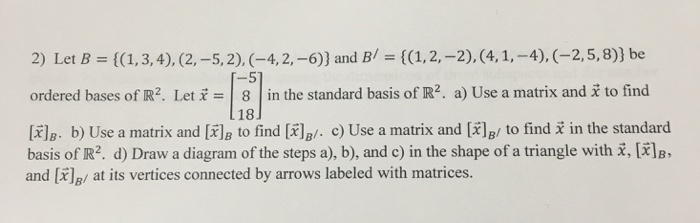2) Let B = {(1, 3, 4), (2,-5,2), (-4,2-6)) and B/-(( 1, 2,-2), (4, 1,-4), (-2, 5, 8)) be 5 ordered bases of R2. Let x = | 8 | in the standard basis of R2. a) Use a matrix and x to find L18 ]B. b) Use a matrix and [X]B to find [x)B/. c) Use a matrix and [X]B/ to find x in the standard basis of R2, d) Draw a diagram of the steps a), b), and...